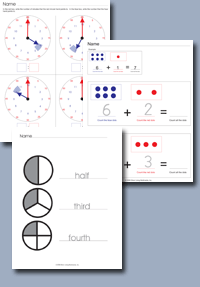﻿ Common Core Standards - Ratios and Proportional Relationships - Grade 6Adaptive Worksheets

Helping Every Child to Succeed

toll-free (888) 777-0876
fax (888) 777-0875

## Ratios and Proportional Relationships - Grade 6

### Understand ratio concepts and use ratio reasoning to solve problems.

• CCSS.MATH.CONTENT.6.RP.A.1
Understand the concept of a ratio and use ratio language to describe a ratio relationship between two quantities. For example, "The ratio of wings to beaks in the bird house at the zoo was 2:1, because for every 2 wings there was 1 beak." "For every vote candidate A received, candidate C received nearly three votes."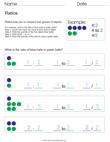Ratio Relationships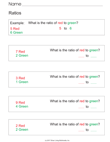Ratio Basics

• CCSS.MATH.CONTENT.6.RP.A.2
Understand the concept of a unit rate a/b associated with a ratio a:b with b ≠ 0, and use rate language in the context of a ratio relationship. For example, "This recipe has a ratio of 3 cups of flour to 4 cups of sugar, so there is 3/4 cup of flour for each cup of sugar, so there is 3/4 cup of flour for each cup of sugar."  or  "We paid \$75 for 15 hamburgers, wich is a rate of \$5 per hamburger."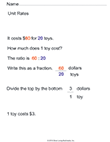Unit Rates with Price Per 1 ItemUnit Rate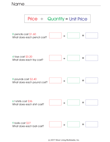Unit Cost
• CCSS.MATH.CONTENT.6.RP.A.3
Use ratio and rate reasoning to solve real-world and mathematical problems, e.g., by reasoning about tables of equivalent ratios, tape diagrams, double number line diagrams, or equations.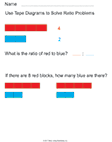Basic Tape Diagrams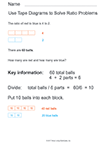Tape Diagrams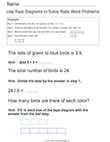Ratio Word Problems with Tape Diagrams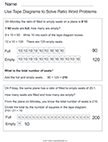More Word Problems with Tape Diagrams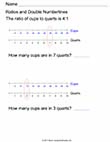Double Number Lines
• CCSS.MATH.CONTENT.6.RP.A.3.A
Make tables of equivalent ratios relating quantities with whole-number measurements, find missing values in the tables, and plot the pairs of values on the coordinate plane. Use tables to compare ratios.
• CCSS.MATH.CONTENT.6.RP.A.3.B
Solve unit rate problems including those involving unit pricing and constant speed. For example, if it took 7 hours to mow 4 lawns, then at that rate, how many lawns could be mowed in 35 hours? At what rate were lawns being mowed?Unit Rate / Constant Speed
• CCSS.MATH.CONTENT.6.RP.A.3.C
Find a percent of a quantity as a rate per 100 (e.g., 30% of a quantity means 30/100 times the quantity); solve problems involving finding the whole, given a part and the percent.
• CCSS.MATH.CONTENT.6.RP.A.3.D
Use ratio reasoning to convert measurement units; manipulate and transform units appropriately when multiplying or dividing quantities.